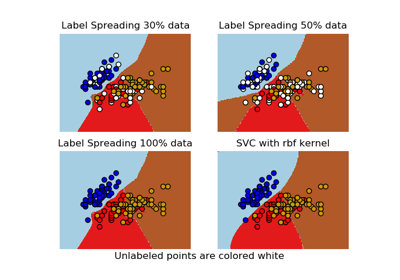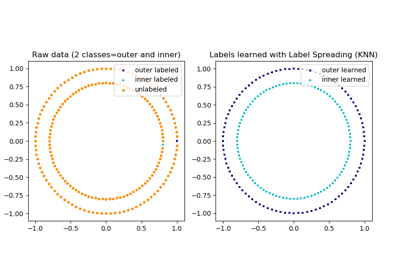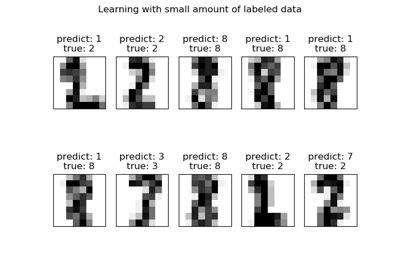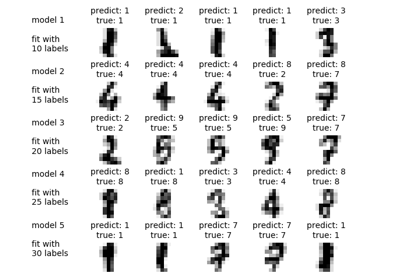# sklearn.semi_supervised.LabelSpreading¶

class sklearn.semi_supervised.LabelSpreading(kernel='rbf', gamma=20, n_neighbors=7, alpha=0.2, max_iter=30, tol=0.001, n_jobs=None)[source]

This model is similar to the basic Label Propagation algorithm, but uses affinity matrix based on the normalized graph Laplacian and soft clamping across the labels.

Read more in the User Guide.

Parameters
kernel{‘knn’, ‘rbf’, callable}

String identifier for kernel function to use or the kernel function itself. Only ‘rbf’ and ‘knn’ strings are valid inputs. The function passed should take two inputs, each of shape [n_samples, n_features], and return a [n_samples, n_samples] shaped weight matrix

gammafloat

parameter for rbf kernel

n_neighborsinteger > 0

parameter for knn kernel

alphafloat

Clamping factor. A value in (0, 1) that specifies the relative amount that an instance should adopt the information from its neighbors as opposed to its initial label. alpha=0 means keeping the initial label information; alpha=1 means replacing all initial information.

max_iterinteger

maximum number of iterations allowed

tolfloat

Convergence tolerance: threshold to consider the system at steady state

n_jobsint or None, optional (default=None)

The number of parallel jobs to run. None means 1 unless in a joblib.parallel_backend context. -1 means using all processors. See Glossary for more details.

Attributes
X_array, shape = [n_samples, n_features]

Input array.

classes_array, shape = [n_classes]

The distinct labels used in classifying instances.

label_distributions_array, shape = [n_samples, n_classes]

Categorical distribution for each item.

transduction_array, shape = [n_samples]

Label assigned to each item via the transduction.

n_iter_int

Number of iterations run.

LabelPropagation

Unregularized graph based semi-supervised learning

References

Dengyong Zhou, Olivier Bousquet, Thomas Navin Lal, Jason Weston, Bernhard Schoelkopf. Learning with local and global consistency (2004) http://citeseer.ist.psu.edu/viewdoc/summary?doi=10.1.1.115.3219

Examples

>>> import numpy as np
>>> from sklearn import datasets
>>> rng = np.random.RandomState(42)
>>> random_unlabeled_points = rng.rand(len(iris.target)) < 0.3
>>> labels = np.copy(iris.target)
>>> labels[random_unlabeled_points] = -1
>>> label_prop_model.fit(iris.data, labels)


Methods

 fit(self, X, y) Fit a semi-supervised label propagation model based get_params(self[, deep]) Get parameters for this estimator. predict(self, X) Performs inductive inference across the model. predict_proba(self, X) Predict probability for each possible outcome. score(self, X, y[, sample_weight]) Return the mean accuracy on the given test data and labels. set_params(self, \*\*params) Set the parameters of this estimator.
__init__(self, kernel='rbf', gamma=20, n_neighbors=7, alpha=0.2, max_iter=30, tol=0.001, n_jobs=None)[source]

Initialize self. See help(type(self)) for accurate signature.

fit(self, X, y)[source]

Fit a semi-supervised label propagation model based

All the input data is provided matrix X (labeled and unlabeled) and corresponding label matrix y with a dedicated marker value for unlabeled samples.

Parameters
Xarray-like of shape (n_samples, n_features)

A {n_samples by n_samples} size matrix will be created from this

yarray_like, shape = [n_samples]

n_labeled_samples (unlabeled points are marked as -1) All unlabeled samples will be transductively assigned labels

Returns
selfreturns an instance of self.
get_params(self, deep=True)[source]

Get parameters for this estimator.

Parameters
deepbool, default=True

If True, will return the parameters for this estimator and contained subobjects that are estimators.

Returns
paramsmapping of string to any

Parameter names mapped to their values.

predict(self, X)[source]

Performs inductive inference across the model.

Parameters
Xarray-like of shape (n_samples, n_features)
Returns
yarray_like, shape = [n_samples]

Predictions for input data

predict_proba(self, X)[source]

Predict probability for each possible outcome.

Compute the probability estimates for each single sample in X and each possible outcome seen during training (categorical distribution).

Parameters
Xarray-like of shape (n_samples, n_features)
Returns
probabilitiesarray, shape = [n_samples, n_classes]

Normalized probability distributions across class labels

score(self, X, y, sample_weight=None)[source]

Return the mean accuracy on the given test data and labels.

In multi-label classification, this is the subset accuracy which is a harsh metric since you require for each sample that each label set be correctly predicted.

Parameters
Xarray-like of shape (n_samples, n_features)

Test samples.

yarray-like of shape (n_samples,) or (n_samples, n_outputs)

True labels for X.

sample_weightarray-like of shape (n_samples,), default=None

Sample weights.

Returns
scorefloat

Mean accuracy of self.predict(X) wrt. y.

set_params(self, **params)[source]

Set the parameters of this estimator.

The method works on simple estimators as well as on nested objects (such as pipelines). The latter have parameters of the form <component>__<parameter> so that it’s possible to update each component of a nested object.

Parameters
**paramsdict

Estimator parameters.

Returns
selfobject

Estimator instance.

## Examples using sklearn.semi_supervised.LabelSpreading¶